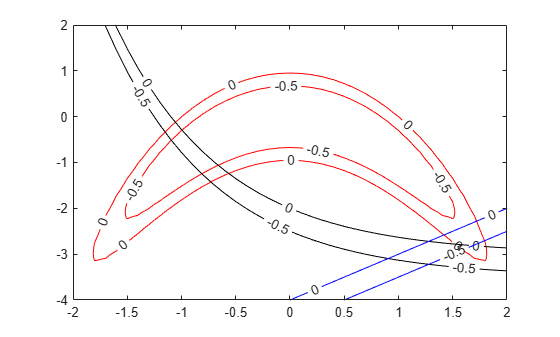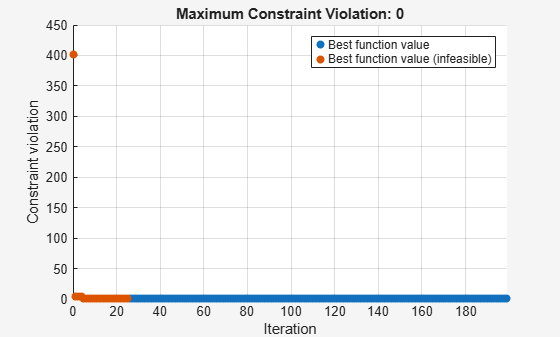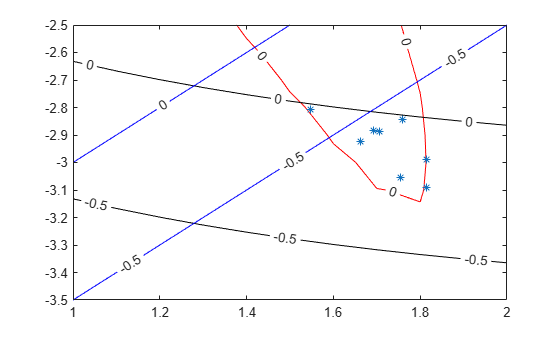# Solve Feasibility Problem

Some problems require you to find a point that satisfies all constraints, with no objective function to minimize. For example, suppose that you have the following constraints:

`$\begin{array}{l}\left(y+{x}^{2}{\right)}^{2}+0.1{y}^{2}\le 1\\ y\le \mathrm{exp}\left(-x\right)-3\\ y\le x-4.\end{array}$`

Do any points $\left(x,y\right)$ satisfy the constraints? To find out, write a function that returns the constraints in a structure field `Ineq`. Write the constraints in terms of a two-element vector $x=\left({x}_{1},{x}_{2}\right)$ instead of $\left(x,y\right)$. Write each inequality as a function $c\left(x\right)$, meaning the inequalities $c\left(x\right)\le 0$, by subtracting the right side of each inequality from both sides. To enable plotting, write the function in a vectorized manner, where each row represents one point. The code for this helper function, named `objconstr`, appears at the end of this example.

Plot the points where the three functions satisfy equalities for $-2\le x\le 2$ and $-4\le y\le 2$, and indicate the inequalities by plotting level lines for function values equal to –1/2.

```[XX,YY] = meshgrid(-2:0.1:2,-4:0.1:2); ZZ = objconstr([XX(:),YY(:)]).Ineq; ZZ = reshape(ZZ,[size(XX),3]); h = figure; ax = gca; contour(ax,XX,YY,ZZ(:,:,1),[-1/2 0],'r','ShowText','on'); hold on contour(ax,XX,YY,ZZ(:,:,2),[-1/2 0],'k','ShowText','on'); contour(ax,XX,YY,ZZ(:,:,3),[-1/2 0],'b','ShowText','on'); hold off```The plot shows that feasible points exist near [1.75,–3].

Set lower bounds of –5 and upper bounds of 3, and solve the problem using `surrogateopt`.

```rng(1) % For reproducibility lb = [-5,-5]; ub = [3,3]; [x,fval,exitflag,output,trials] = surrogateopt(@objconstr,lb,ub)``````surrogateopt stopped because it exceeded the function evaluation limit set by 'options.MaxFunctionEvaluations'. ```
```x = 1×2 1.6307 -2.8147 ```
```fval = 1x0 empty double row vector ```
```exitflag = 0 ```
```output = struct with fields: elapsedtime: 17.8575 funccount: 200 constrviolation: -0.0105 ineq: [-0.1836 -0.0105 -0.4453] rngstate: [1x1 struct] message: 'surrogateopt stopped because it exceeded the function evaluation limit set by ...' ```
```trials = struct with fields: X: [200x2 double] Ineq: [200x3 double] ```

Check the feasibility at the returned solution `x`.

`disp(output.ineq)`
``` -0.1836 -0.0105 -0.4453 ```

Equivalently, evaluate the function `objconstr` at the returned solution `x`.

`disp(objconstr(x).Ineq)`
``` -0.1836 -0.0105 -0.4453 ```

Equivalently, examine the `Ineq` field in the `trials` structure for the solution `x`. First, find the index of `x` in the `trials.X` field.

```indx = ismember(trials.X,x,'rows'); disp(trials.Ineq(indx,:))```
``` -0.1836 -0.0105 -0.4453 ```

All constraint function values are negative, indicating that the point `x` is feasible.

View the feasible points evaluated by `surrogateopt`.

```opts = optimoptions("surrogateopt"); indx = max(trials.Ineq,[],2) <= opts.ConstraintTolerance; % Indices of feasible points figure(h); hold on plot(trials.X(indx,1),trials.X(indx,2),'*') xlim([1 2]) ylim([-3.5 -2.5]) hold off```This code creates the `objconstr` helper function.

```function f = objconstr(x) c(:,1) = (x(:,2) + x(:,1).^2).^2 + 0.1*x(:,2).^2 - 1; c(:,2) = x(:,2) - exp(-x(:,1)) + 3; c(:,3) = x(:,2) - x(:,1) + 4; f.Ineq = c; end```